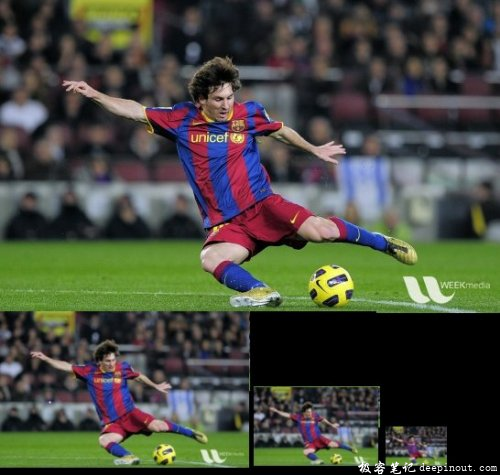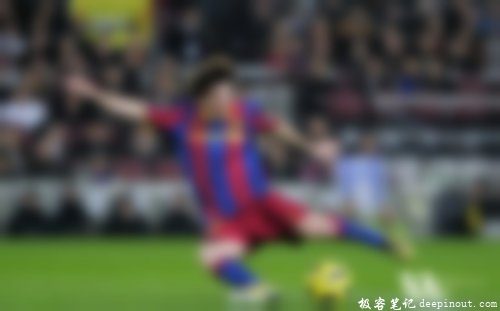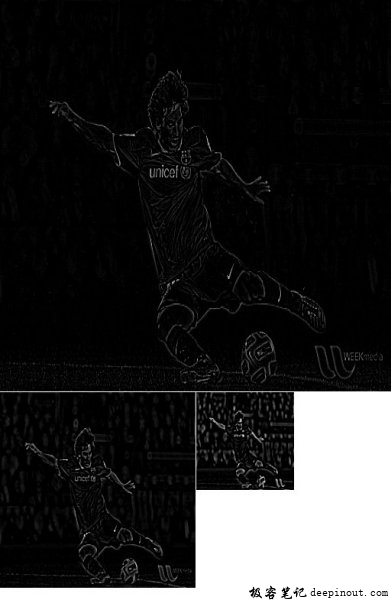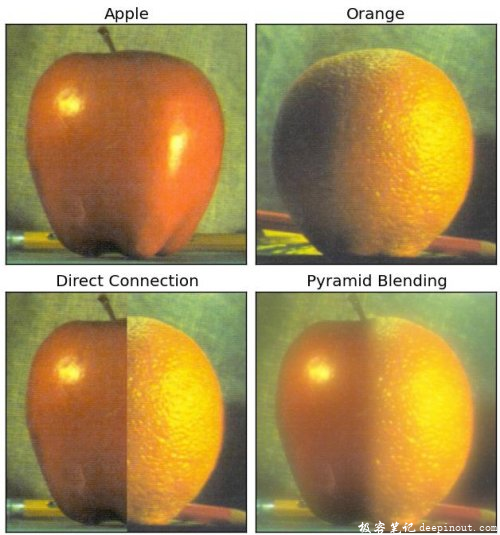# OpenCV-Python图像金字塔

• 我们将了解图像金字塔。
• 我们将使用图像金字塔创建一个新的水果，“Orapple”
• 我们将看到这些功能： cv.pyrUp（）cv.pyrDown（）

## 图像金字塔理论

img = cv.imread('messi5.jpg')lower_reso = cv.pyrDown(higher_reso)higher_reso2 = cv.pyrUp(lower_reso)## 使用金字塔的图像混合1. 加载苹果和橙色的两个图像
2. 找到苹果和橙色的高斯金字塔（在这个例子中，级别数是 6）
3. 从高斯金字塔，找到他们的拉普拉斯金字塔
4. 现在加入左半部分的苹果和右半部分的拉普拉斯金字塔
5. 最后，从这个联合图像金字塔，重建原始图像。

import cv2 as cv
import numpy as np,sys
# generate Gaussian pyramid for A
G = A.copy()
gpA = [G]
for i in xrange(6):
G = cv.pyrDown(G)
gpA.append(G)
# generate Gaussian pyramid for B
G = B.copy()
gpB = [G]
for i in xrange(6):
G = cv.pyrDown(G)
gpB.append(G)
# generate Laplacian Pyramid for A
lpA = [gpA]
for i in xrange(5,0,-1):
GE = cv.pyrUp(gpA[i])
L = cv.subtract(gpA[i-1],GE)
lpA.append(L)
# generate Laplacian Pyramid for B
lpB = [gpB]
for i in xrange(5,0,-1):
GE = cv.pyrUp(gpB[i])
L = cv.subtract(gpB[i-1],GE)
lpB.append(L)
# Now add left and right halves of images in each level
LS = []
for la,lb in zip(lpA,lpB):
rows,cols,dpt = la.shape
ls = np.hstack((la[:,0:cols/2], lb[:,cols/2:]))
LS.append(ls)
# now reconstruct
ls_ = LS
for i in xrange(1,6):
ls_ = cv.pyrUp(ls_)